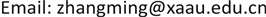OJNSOpen Journal of Nature Science2330-1724Scientific Research Publishing10.12677/OJNS.2018.65049OJNS-26542OJNS20180500000_58414359.pdf数学与物理 地球与环境 信息通讯 生命科学 化学与材料 Wald测试粒子近似方法在弱的宇宙监督假设中的应用 Application of Wald Test Particle Approximation Method in the Weak Cosmic Censorship Conjecture 1*21null西安航空学院理学院，陕西 西安* E-mail:zhangming@xaau.edu.cn(张明);220820180605383390© Copyright 2014 by authors and Scientific Research Publishing Inc. 2014This work is licensed under the Creative Commons Attribution International License (CC BY). http://creativecommons.org/licenses/by/4.0/

Wald测试粒子近似方法在弱的宇宙监督假设中的应用<sup> </sup>Copyright © 2018 by authors and Hans Publishers Inc.

This work is licensed under the Creative Commons Attribution International License (CC BY).

http://creativecommons.org/licenses/by/4.0/1. 引言

2. Wald测试粒子近似方法介绍

Kerr-Newman  黑洞的线元为

d s 2 = − ( 1 − 2 M r − Q 2 ρ 2 ) d t 2 + ρ 2 Δ d r 2 + ρ 2 d θ 2     + [ ( r 2 + a 2 ) sin 2 θ + ( 2 M r − Q 2 ) a 2 sin 4 θ ρ 2 ] d ϕ 2     − 2 ( 2 M r − Q 2 ) a sin 2 θ ρ 2 d t d ϕ (1)

r + = M + M 2 − Q 2 − a 2 (2)

M 2 = Q 2 + a 2 (3)

Kerr-Newman黑洞拥有事件视界必须要满足条件

M 2 ≥ Q 2 + a 2 (4)

M 2 < Q 2 + a 2 (5)

( M + E ) 2 < ( a M + L M + E ) 2 + ( q + Q ) 2 (6)

E < Q q M + a L M 2 + a 2 (7)

E = g t ϕ g ϕ ϕ ( q A ϕ − L ) − q A t + ( g t ϕ 2 − g ϕ ϕ g t t g ϕ ϕ 2 ) ( ( L − q A ϕ ) 2 + m 2 g ϕ ϕ ( 1 + g r r r ˙ 2 + g θ θ θ ˙ 2 ) )   (8)

E > g t ϕ g ϕ ϕ ( q A ϕ − L ) − q A t (9)

E min < E < E max (10)

3. Wald测试粒子方法研究综述3.1. 考虑高阶修正后极端Kerr-Newman黑洞的视界破坏

Wald假想试验发现极端的Kerr-Newman黑洞通过捕获一个测试粒子是无法出现裸奇点的，Wald在计算时对粒子一些参数作了线性近似。后来有研究者指出  ，在不作线性近似的情况下，测试粒子是可以破坏一个极端的Kerr-Newman黑洞的，其所允许的破坏黑洞的粒子的能量范围很窄。定义新参量W

W = ( M + E ) 2 (11)

W 2 − ( Q + q ) 2 − ( a M + L ) 2 < 0 (12)

W 1 < W < W 2 , (13)

W 1 , 2 = ( Q + q ) 2 ± ( Q + q ) 4 + 4 ( a M + L ) 2 2 (14)

W > ( a L + q Q M a 2 + M 2 + M ) 2 ≡ W 3 (15)

s ≡ W 2 − W 3 = ( Q + q ) 2 + ( Q + q ) 4 + 4 ( a M + L ) 2 2 − ( a L + q Q M a 2 + M 2 + M ) 2 > 0 (16)

3.2. 近极端RN黑洞视界破坏

Hubeny指出  ，通过向一个近极端的RN黑洞投入一个带电的粒子，黑洞的视界可以被破坏。其中粒子的最小能量E

E > q Q r + (17)

r + = M + M 2 − Q 2 (18)

E < Q + q − M (19)

q > r + ( M − Q r + − Q ) = r + − Q 2 (20)

m < Q − E 2 + 2 M Q E q − q 2 M 2 − Q 2 (21)

3.3. 近极端Kerr黑洞视界面的破坏

Jacobson和Sotiriou研究发现  ，一个近极端的Kerr黑洞视界可以通过捕获一个有角动量的粒子被破坏。文章中定义测试粒子带有 δ E 的能量和 δ J 的角动量。为了使测试粒子能够掉入黑洞，其角动量必须满足条件

δ J > δ J min = ( M 2 − J ) + 2 M δ E + ( δ E ) 2 (22)

δ J < δ J max = 2 M r + a δ E (23)

3.4. 近极端Kerr-Newman黑洞视界面的破坏

E < E max = Q e M + a L M 2 + a 2 − M 3 2 ( M 2 + a 2 ) ( δ M ) 2 (24)

E ≥ = A − B ( δ M ) − ( ( 2 + sin 2 α ) A − 4 B 2 + 2 cos 2 α ) ( δ M ) 2 (25)

A = ( L / M ) cos α + e sin α 1 + cos 2 α ≥ 0 (26)

B = ( L / M ) cos α + e sin 3 α ( 1 + cos 2 α ) 2 ≥ 0 (27)

a = M 2 − δ 2 cos α (28)

Q = M 2 − δ 2 sin α (29)

3.5. 更多的研究进展